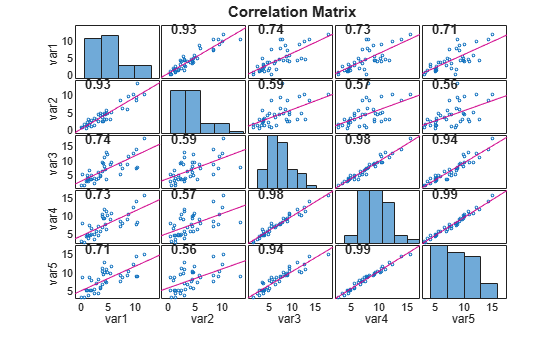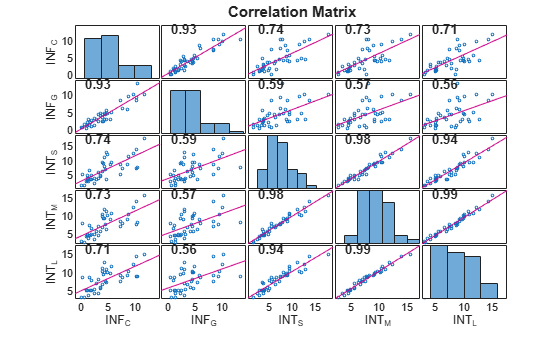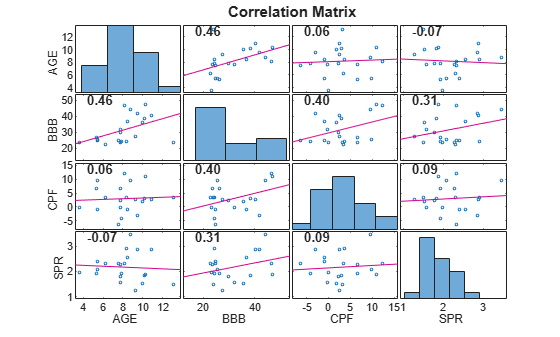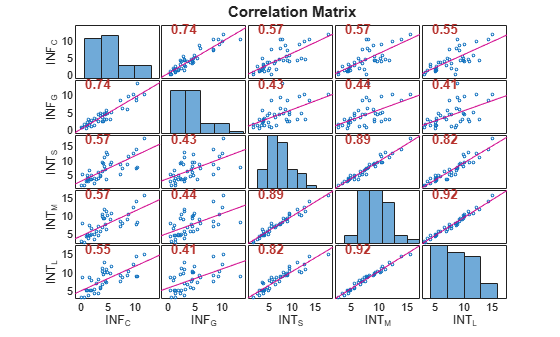# corrplot

Plot variable correlations

## Syntax

``````[R,PValue] = corrplot(X)``````
``[R,PValue] = corrplot(Tbl)``
``[___] = corrplot(___,Name=Value)``
``corrplot(___)``
``corrplot(ax,___)``
``````[___,H] = corrplot(___)``````

## Description

example

``````[R,PValue] = corrplot(X)``` plots Pearson's correlation coefficients between all pairs of variables in the matrix of time series data `X`. The plot is a `numVars`-by-`numVars` grid, where `numVars` is the number of time series variables (columns) in `X`, including the following subplots: Each off diagonal subplot contains a scatterplot of a pair of variables with a least-squares reference line, the slope of which is equal to the displayed correlation coefficient.Each diagonal subplot contains the distribution of a variable as a histogram. Also, the function returns the correlation matrix in the plots `R` and a matrix of p-values `PValue` for testing the null hypothesis that each pair of coefficients is not correlated against the alternative hypothesis of a nonzero correlation.```

example

````[R,PValue] = corrplot(Tbl)` plots the Pearson's correlation coefficients between all pairs of variables in the table or timetable `Tbl`, and also returns tables for the correlation matrix `R` and matrix of p-values `PValue`.To select a subset of variables in `Tbl`, for which to plot the correlation matrix, use the `DataVariables` name-value argument.```

example

````[___] = corrplot(___,Name=Value)` specifies options using one or more name-value arguments in addition to any of the input argument combinations in previous syntaxes. `corrplot` returns the output argument combination for the corresponding input arguments. For example, `corrplot(Tbl,Type="Spearman",TestR="on",DataVariables=1:5)` computes Spearman’s rank correlation coefficient for the first 5 variables of the table `Tbl` and tests for significant correlation coefficients.```

example

``corrplot(___)` plots the correlation matrix.`
````corrplot(ax,___)` plots on the axes specified by `ax` instead of the current axes (`gca`). `ax` can precede any of the input argument combinations in the previous syntaxes.```
``````[___,H] = corrplot(___)``` plots the diagnostics of the input series and additionally returns handles to plotted graphics objects `H`. Use elements of `H` to modify properties of the plot after you create it.```

## Examples

collapse all

Plot and return Pearson's correlation coeffifients between pairs of time series using the default options of `corrplot`. Input the time series data as a numeric matrix.

Load data of Canadian inflation and interest rates `Data_Canada.mat`, which contains the series in the matrix `Data`.

`load Data_Canada`

Plot and return the correlation matrix between all pairs of variables in the data.

`R = corrplot(Data)````R = 5×5 1.0000 0.9266 0.7401 0.7287 0.7136 0.9266 1.0000 0.5908 0.5716 0.5556 0.7401 0.5908 1.0000 0.9758 0.9384 0.7287 0.5716 0.9758 1.0000 0.9861 0.7136 0.5556 0.9384 0.9861 1.0000 ```

The correlation plot shows that the short-term, medium-term, and long-term interest rates are highly correlated.

Plot correlations between time series, which are variables in a table, using default options. Return a table of pairwise correlations and a table of corresponding significance-test $\mathit{p}$-values.

Load data of Canadian inflation and interest rates `Data_Canada.mat`. Convert the table `DataTable` to a timetable.

```load Data_Canada dates = datetime(dates,ConvertFrom="datenum"); TT = table2timetable(DataTable,RowTimes=dates); TT.Observations = [];```

Plot and return the correlation matrix, with corresponding significance-test $\mathit{p}$-values, between all pairs of variables in the data

`[R,PValue] = corrplot(TT)````R=5×5 table INF_C INF_G INT_S INT_M INT_L _______ _______ _______ _______ _______ INF_C 1 0.92665 0.74007 0.72867 0.7136 INF_G 0.92665 1 0.59077 0.57159 0.55557 INT_S 0.74007 0.59077 1 0.9758 0.93843 INT_M 0.72867 0.57159 0.9758 1 0.98609 INT_L 0.7136 0.55557 0.93843 0.98609 1 ```
```PValue=5×5 table INF_C INF_G INT_S INT_M INT_L __________ __________ __________ __________ __________ INF_C 1 3.6657e-18 3.2113e-08 6.6174e-08 1.6318e-07 INF_G 3.6657e-18 1 4.7739e-05 9.4769e-05 0.00016278 INT_S 3.2113e-08 4.7739e-05 1 2.3206e-27 1.3408e-19 INT_M 6.6174e-08 9.4769e-05 2.3206e-27 1 5.1602e-32 INT_L 1.6318e-07 0.00016278 1.3408e-19 5.1602e-32 1 ```

`corrplot` returns the correlation matrix and corresponding matrix of $\mathit{p}$-values in tables `R` and `PValue`, respectively.

By default, `corrplot` computes correlations between all pairs of variables in the input table. To select a subset of variables from an input table, set the `DataVariables` option.

Plot the correlation matrix for selected time series.

Load the credit default data set `Data_CreditDefaults.mat`. The table `DataTable` contains the default rate of investment-grade corporate bonds series (`IGD`, the response variable) and several predictor variables.

`load Data_CreditDefaults`

Consider a multiple regression model for the default rate that includes an intercept term.

Include a variable in the table of data that represents the intercept in the design matrix (that is, a column of ones). Place the intercept variable at the beginning of the table.

```Const = ones(height(DataTable),1); DataTable = addvars(DataTable,Const,Before=1);```

Create a variable that contains all predictor variable names.

```varnames = DataTable.Properties.VariableNames; prednames = varnames(varnames ~= "IGD");```

Graph a correlation plot of all predictor variables except for the intercept dummy variable.

`corrplot(DataTable,DataVariables=prednames(2:end));`The predictor `BBB` is moderately linearly associated with the other predictors, while all other predictors appear unassociated with each other.

Plot Kendall's rank correlations between multiple time series. Conduct a hypothesis test to determine which correlations are significantly different from zero.

`load Data_Canada`

Plot the Kendall's rank correlation coefficients between all pairs of variables. Identify which correlations are significantly different from zero by conducting hypothesis tests.

`corrplot(DataTable,Type="Kendall",TestR="on")`The correlation coefficients highlighted in red indicate which pairs of variables have correlations significantly different from zero. For these time series, all pairs of variables have correlations significantly different from zero.

Test for correlations greater than zero between multiple time series.

Load data on Canadian inflation and interest rates `Data_Canada.mat`.

`load Data_Canada`

Return the pairwise Pearson's correlations and corresponding $\mathit{p}$-values for testing the null hypothesis of no correlation against the right-tailed alternative that the correlations are greater than zero.

`[R,PValue] = corrplot(DataTable,Tail="right");``PValue`
```PValue=5×5 table INF_C INF_G INT_S INT_M INT_L __________ __________ __________ __________ __________ INF_C 1 1.8329e-18 1.6056e-08 3.3087e-08 8.1592e-08 INF_G 1.8329e-18 1 2.3869e-05 4.7384e-05 8.1392e-05 INT_S 1.6056e-08 2.3869e-05 1 1.1603e-27 6.7041e-20 INT_M 3.3087e-08 4.7384e-05 1.1603e-27 1 2.5801e-32 INT_L 8.1592e-08 8.1392e-05 6.7041e-20 2.5801e-32 1 ```

The output `PValue` has pairwise $\mathit{p}$-values all less than the default 0.05 significance level, indicating that all pairs of variables have correlation significantly greater than zero.

## Input Arguments

collapse all

Time series data, specified as a `numObs`-by-`numVars` numeric matrix. Each column of `X` corresponds to a variable, and each row corresponds to an observation.

Data Types: `double`

Time series data, specified as a table or timetable with `numObs` rows. Each row of `Tbl` is an observation.

Specify `numVars` variables to include in the diagnostics computations by using the `DataVariables` argument. The selected variables must be numeric.

Axes on which to plot, specified as an `Axes` object.

By default, `corrplot` plots to the current axes (`gca`).

`corrplot` does not support `UIAxes` targets.

### Name-Value Arguments

Specify optional pairs of arguments as `Name1=Value1,...,NameN=ValueN`, where `Name` is the argument name and `Value` is the corresponding value. Name-value arguments must appear after other arguments, but the order of the pairs does not matter.

Before R2021a, use commas to separate each name and value, and enclose `Name` in quotes.

Example: `corrplot(Tbl,Type="Spearman",TestR="on",DataVariables=1:5)` computes Spearman’s rank correlation coefficient for the first 5 variables of the table `Tbl` and tests for significant correlation coefficients.

Correlation coefficient to compute, specified as a value in this table.

ValueDescription
`"Pearson"`Pearson’s linear correlation coefficient
`"Kendall"`Kendall’s rank correlation coefficient (τ)
`"Spearman"`Spearman’s rank correlation coefficient (ρ)

Example: `Type="Kendall"`

Data Types: `char` | `string`

Option for handling rows in the input time series data that contain `NaN` values, specified as a value in this table.

ValueDescription
`"all"`Use all rows, regardless of any `NaN` entries.
`"complete"`Use only rows that do not contain `NaN` entries.
`"pairwise"`Use rows that do not contain `NaN` entries in column (variable) i or j to compute R(i,j).

Example: `Rows="complete"`

Data Types: `char` | `string`

Alternative hypothesis Ha used to compute the p-values `PValue`, specified as a value in this table.

ValueDescription
`"both"`Ha: Correlation is not zero.
`"right"`Ha: Correlation is greater than zero.
`"left"`Ha: Correlation is less than zero.

Example: `Tail="left"`

Data Types: `char` | `string`

Unique variable names used in the plots, specified as a string vector or cell vector of strings of a length `numVars`. `VarNames(j)` specifies the name to use for variable `X(:,j)` or `DataVariables(j)`.

• If the input time series data is the matrix `X`, the default is `{'var1','var2',...}`.

• If the input time series data is the table or timetable `Tbl`, the default is `Tbl.Properties.VariableNames`.

Example: `VarNames=["Const" "AGE" "BBD"]`

Data Types: `char` | `cell` | `string`

Flag for testing whether correlations are significant, specified as a value in this table.

ValueDescription
`"on"``corrplot` highlights significant correlations in the correlation matrix plot using red font.
`"off"`All correlations in the correlation matrix plot have black font.

Example: `TestR="on"`

Data Types: `char` | `string`

Significance level for correlation tests, specified as a scalar in the interval [0,1].

Example: `Alpha=0.01`

Data Types: `double`

Variables in `Tbl` for which `corrplot` includes in the correlation matrix plot, specified as a string vector or cell vector of character vectors containing variable names in `Tbl.Properties.VariableNames`, or an integer or logical vector representing the indices of names. The selected variables must be numeric.

Example: `DataVariables=["GDP" "CPI"]`

Example: `DataVariables=[true true true false]` or `DataVariables=1:3` selects the first through third table variables.

Data Types: `double` | `logical` | `char` | `cell` | `string`

## Output Arguments

collapse all

Correlations between pairs of variables in the input time series data that are displayed in the plots, returned as one of the following quantities:

• `numVars`-by-`numVars` numeric matrix when you supply the input `X`.

• `numVars`-by-`numVars` table when you supply the input `Tbl`, where `numVars` is the selected number of variables in the `DataVariables` argument.

p-values corresponding to significance tests on the elements of `R`, returned as one of the following quantities:

• `numVars`-by-`numVars` numeric matrix when you supply the input `X`.

• `numVars`-by-`numVars` table when you supply the input `Tbl`, where the variables specified by the `DataVariables` argument determines `numVars` and the names of the rows and columns of the output table.

The p-values are used to test the null hypothesis of no correlation against the alternative hypothesis of a nonzero correlation, with test tail specified by the `TestR` argument.

Handles to plotted graphics objects, returned as one of the following quantities:

• `numVars`-by-`numVars` matrix of graphics objects when you supply the input `X`

• `numVars`-by-`numVars` table of graphics objects when you supply the input `Tbl`, where the variables specified by the `DataVariables` argument determines `numVars` and the names of the rows and columns of the output table

`H` contains unique plot identifiers, which you can use to query or modify properties of the plot.

## Tips

• The setting `Rows="pairwise"` (the default) can return a correlation matrix that is not positive definite. The setting `Rows="complete"` returns a positive-definite matrix, but, in general, the estimates are based on fewer observations.

## Algorithms

• `corrplot` computes p-values for Pearson’s correlation by transforming the correlation to create a t-statistic with `numObs` – 2 degrees of freedom. The transformation is exact when the input time series data is normal.

• `corrplot` computes p-values for Kendall’s and Spearman’s rank correlations by using either the exact permutation distributions (for small sample sizes) or large-sample approximations.

• `corrplot` computes p-values for two-tailed tests by doubling the more significant of the two one-tailed p-values.

## Version History

Introduced in R2012a

expand all